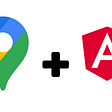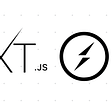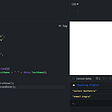# Array

what is array?. An array consists of one or more data. Array is a data structure where we can store multiple data.
Now the question that come to your mind why we need array?. If we have single data we can store easily put in a data variable. But If we have more data what we will do? .we will put multiple data one variable ? .No never it is not possible. So we need array .

If you have a list of items (a list of student names, for example), storing the student name in single variables could look like this:

var student1 = “shakib”

var student2 = “rakib”

var student3= “shibab”

var student4 = “michel”

It doesn’t look good and It will create complexity when creating large application.That's why we need array.

How do we recognize the array in javascript ? It is look like second bracket [ ] . It is called empty array.Anything inside the second bracket it will be array. One way of creating arrays is as follows:
For example of array ,
var name = [ ‘rakib’ , ‘sihab’ , ‘mehedi’] ;
we can store any type of data like boolean,string,integer etc in array.

# parseInt()

parseInt() method return the value a integer number.
example: var num = parseInt(10.44)
console.log(num) // 10
note that parseInte() method only working on number.If you pass a string on this method it is always return NAN

# Math.min() && Math.max()

Math objects can be used to mathematical terms.
Ther are many method of math object.Math.min() && Math.max() one of them.
You can get minumum number from multiple numbers use Math.min() method.
example: Math.min(0, 150, 30, 20, -8); // returns -8

You can get maximum number from multiple numbers use Math.min() method.
example: Math.max(0, 150, 30, 20, -8); // returns 150

# Math.floor()

This function of this method convert to decimal number to integer number.It always returns integer number like parseInt() method
example : Math.floor(10.10)// return 10
even It is 10.99 then it will be returned 10. That means it always returns an integer number.

# Array push()

The push method add a new item to the end of the array and it will change the array length.
example : var name = [ ‘rakib’ , ‘jakib’ , ‘riyan’ , ‘kamil’ , ‘jamil’]
name.push(‘michel)
console.log(name) // result ‘rakib’ , ‘jakib’ , ‘riyan’ , ‘kamil’ , ‘jamil’ , ‘michel’
console.log(name.length) // 6
see the length has been changing 6 and add a new item to the end.

it is the same as Array push() just different is add item at the begining of an array and it will change array length and length will increase.
example:
var name = [ ‘rakib’ , ‘jakib’ , ‘riyan’ , ‘kamil’ , ‘jamil’]
name. unshift(‘michel’)
console.log(name) // result ‘michel’, ‘rakib’ , ‘jakib’ , ‘riyan’ , ‘kamil’ , ‘jamil’
see the length has been changing 6 and add a new item to the beginning.

# Array Pop()

The pop() method remove the item at the end of array and array length will be decrease.
example :
var fruits = [“Banana”, “Orange”, “Apple”, “Mango”];
fruits.pop();
console.log(fruits)//”Banana”, “Orange”, “Apple”
console.log(fruits.length) // 2

# Array find()

The find() method will send the first array based on the condition of an array. If condition will match it will be return first array or true value. It is doesn’t execute empty array .It doesn’t change orginal array.
example:
const ages = [10,11,12,13,14,15,16,17,18,19,20]
const result = ages.find(age => age === 15)
console.log(result) //15

10.Convert string toLowercase && toUpperCase()
if you want to convert string Upper case toLowerCase just follow simple method :-
var str = ‘HELLO PROGRAMMER’
var res = str.toLowerCase() ;
console.log(res) // hello programmer

If you want to convert string lowecase toUpperCase. You need to follow toUpperCase() method.This method same as toLowerCase method.
var str = ‘hello world’
var res = str. toUpperCase();
console.log(res) //HELLO WORLD

--

--

--

Love podcasts or audiobooks? Learn on the go with our new app.

## Image goes here…## JavaScript Algorithms: Meeting Rooms (LeetCode)## React Native Ecommerce App 🛒## Integrating Google Maps in React Native App on iOS## Google Maps in Angular with Marker, Info Window and Marker Clustering.## Deploy Socket.io to Kubernetes - Part 1: Chat Application## PEG away at evaluating expressions in JavaScript## call, apply and bind in JavaScript## Event Loop in JavaScript